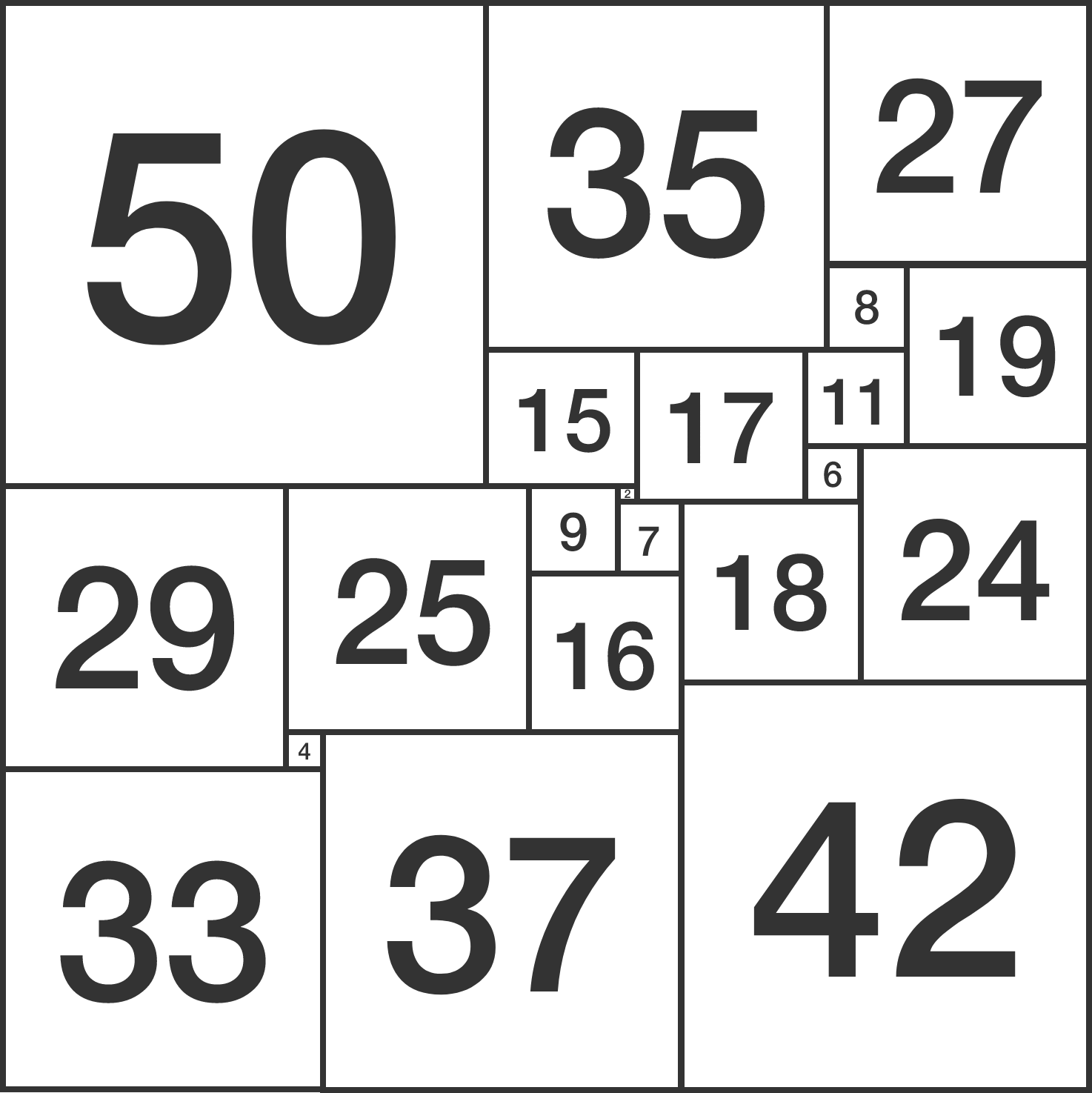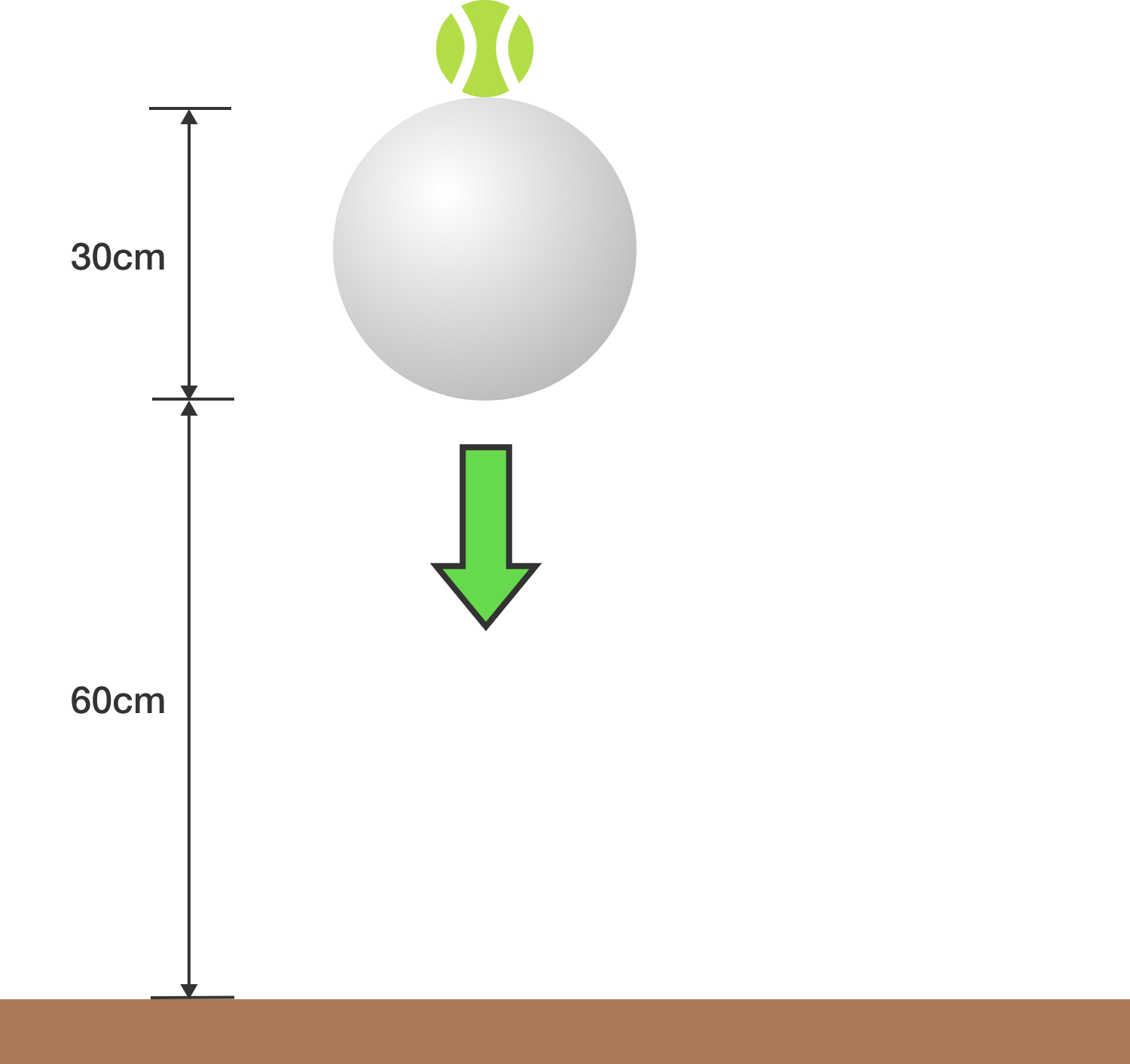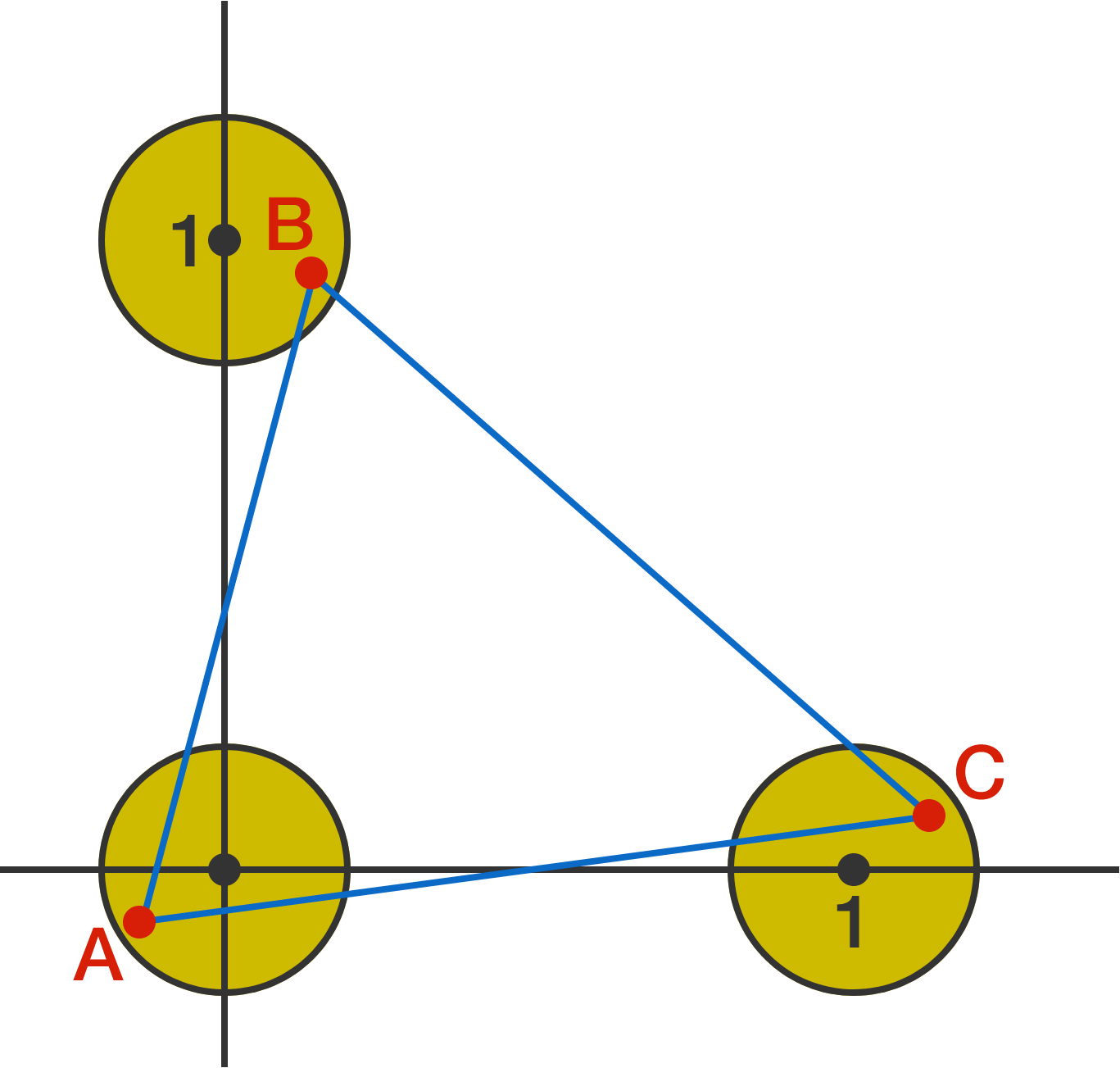# Problems of the Week

Contribute a problemAs shown above, a square can be dissected into squares of distinct integer side lengths.

Is it possible to dissect a cube into cubes of distinct integer side lengths?


Clarification: The number in each square is the side length of that square.A tennis ball rests directly on top of a cannon ball, which has a much larger mass, i.e. $M_\textrm{cannonball} \gg M_\textrm{tennis}.$

When the balls are dropped, to what height (in $\si{\centi\meter}$) will the bottom of the tennis ball bounce?


Details and Assumptions:

• The bottom of the cannon ball starts at a height of $h = \SI{60}{\centi\meter}$ from the ground, and the cannon ball has diameter $d=\SI{30}{\centi\meter}$.
• All collisions are perfectly elastic, and wind resistance is ignored.

$x$ and $y$ are positive numbers such that

$\sqrt { xy } \left( x-y \right) =x+y.$

Find the minimum value of $x+y$ to 2 decimal places.

Let $S(n)$ be the sum of digits of a positive integer $n$ (when written in base 10).

If $S(n) = 4$, find the maximum value of $S\big(n^4\big)$.

Three circles with equal radii are centered at $(1,0), (0,0),$ and $(0,1)$.To 4 decimal places, what is the smallest radius of the circles such that we can pick a point within each circle--like A, B, C in the diagram--and connect them to form an equilateral triangle?

×